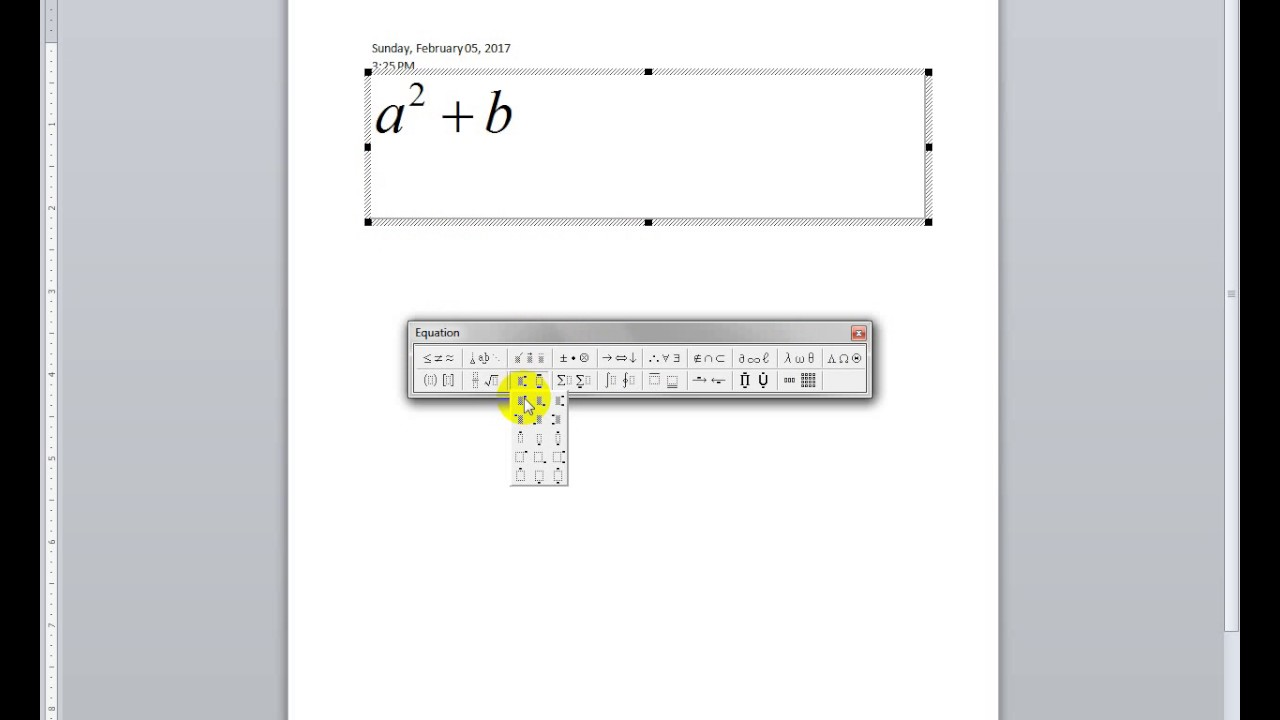Microsoft Equation 3.0 ObjectWhile creating your Word document, you may need to use mathematical expressions. There are times when you may need to write fractions, summations, or insert symbols into your document. The Equation Editor lets you easily choose from a toolbar containing math symbols or templates you may need. This document explains the Equation Editor and how it can be used to create equations.

When you want to insert the Equation object, you must select Insert-Object and select the Microsoft Equation 3.0 in the Object type box. With this, it is serious to insert a lot of Equation object. When you install this add-in, the Equation Editor Button is inserted on the Command bar. Normal Access to Equation Editor.

The Equation Editor lets you insert symbols and build complex equations. Once you open the Equation Editor, a screen appears where you can manage and edit your math equations. The Equation Editor includes a toolbar that has many math symbols (e.g., greater than or less than sign, infinity, equal sign) and templates (e.g., fractions, summations, integrals). Once inserted into your Word document, the math symbols or templates act as objects and can be managed similarly.

After inserting a symbol or template, a box appears surrounding the equation. If you want to edit your equation, double click on the box. This opens the Equation Editor, where you can format your equation appropriately. Once you click the mouse outside of the box, the Equation Editor will disappear.

Opening the Equation Editor

The Equation toolbar has two different rows. The top row includes access to more than 150 different math symbols you can insert, and the bottom row accesses about 120 different templates. When you click a symbol or template button, an options palette appears. From the palette you can select the appropriate symbol or template, inserting it onto the work area in the Equation Editor.

To open the Equation Editor and access the Equation toolbar:

1. In Word, place the insertion point where you want the math symbol or template to appear

2. From the Insert menu, select Object..
The Object dialog box appears.

3. Windows: From the Object type scroll box, select Microsoft Equation 3.0
Macintosh: From the Object type scroll box, select Microsoft Equation

4. Click OK
Windows: The EquationEditor and Equation toolbar appear.
Macintosh: The Equation dialog box appears containing the Equation Editor and Equation toolbar.

Using the Equation Editor

After opening the Equation Editor, you are ready to select a symbol or template. Symbols are single characters (e.g., logic symbols, set theory symbols, Greek letters). Templates are symbols that include spaces in which you can type numbers (e.g., fractions, summations, integrals). Once you select a symbol or template it appears in the work area in the Equation Editor. From this, you can modify your symbols or, if you choose to insert a template, you can create an equation.

1. Open the Equation Editor

2. From the Equation toolbar, click the appropriate button
A palette of options appears.

3. From the palette, click the appropriate symbol or template that you want to insert
The selected symbol or template is inserted into the document.

4. Continue with Creating Equations

Creating Equations

After choosing a symbol or template from the Equation toolbar, you are ready to make it an equation by typing numbers or letters in the appropriate text boxes. Once the template appears in the work area of the Equation Editor, an insertion point automatically appears in one of the outlined text boxes. These text boxes are where you will type in the information for your equation. If you do not wish to start where the insertion point is automatically placed, press [Tab] until you reach the appropriate text box.

NOTE: When working with Macintosh, you must create your equation inside of the Equation dialog box. Once you insert it into your Word document by closing the dialog box, you can not add numbers or letters to it.